Courses

# Test: Second Law of Thermodynamics - 1

## 10 Questions MCQ Test Thermodynamics | Test: Second Law of Thermodynamics - 1

Description
This mock test of Test: Second Law of Thermodynamics - 1 for Mechanical Engineering helps you for every Mechanical Engineering entrance exam. This contains 10 Multiple Choice Questions for Mechanical Engineering Test: Second Law of Thermodynamics - 1 (mcq) to study with solutions a complete question bank. The solved questions answers in this Test: Second Law of Thermodynamics - 1 quiz give you a good mix of easy questions and tough questions. Mechanical Engineering students definitely take this Test: Second Law of Thermodynamics - 1 exercise for a better result in the exam. You can find other Test: Second Law of Thermodynamics - 1 extra questions, long questions & short questions for Mechanical Engineering on EduRev as well by searching above.
QUESTION: 1

Solution:
QUESTION: 2

Solution:
QUESTION: 3

### If a heat engine attains 100% thermal efficiency, it violates

Solution:

Such a heat engine is PMM2 which is impossible. It violates kelvin-Plank statement

QUESTION: 4

A refrigerator and a heat pump operate between the same temperature limits. If the COP of the refrigerator is 4, the COP of the heat pump would be

Solution: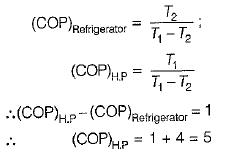QUESTION: 5

If the temperature of the source is increased keeping sink temperature fixed, the efficiency of the Carnot engine

Solution: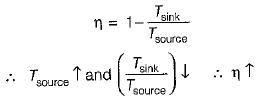QUESTION: 6

The more effective way of increasing the efficiency of a Carnot engine is to

Solution: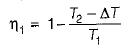(decreasing lower temperature by ΔT)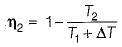(increasing higher temperature by ΔT)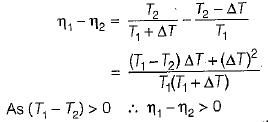So, the more effective way to increase the cycle efficiency is to decrease lower temperature.

QUESTION: 7

A Carnot engine operates between reservoirs at 20°C and 200°C. If 10 kW of power is produced, the rejected heat rate is nearest

Solution: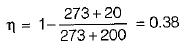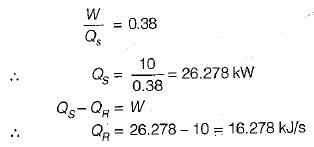QUESTION: 8

An inventor invents a thermal engine that operates between ocean layers at 27°C and 10°C. It produces 10 kW and discharges 9900 kJ/min of heat. Such an enigne is

Solution: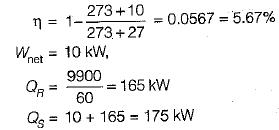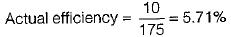Actual efficiency is more than the 2nd law efficiency which is impossible.

QUESTION: 9

If the time taken by a system to execute a process through a finite gradient is infinitely large, the process

Solution:
QUESTION: 10

“Heat cannot be transported from a system at low temperature to another system at high temperature without the aid of external agency”. This statement of second law is attributed to

Solution: